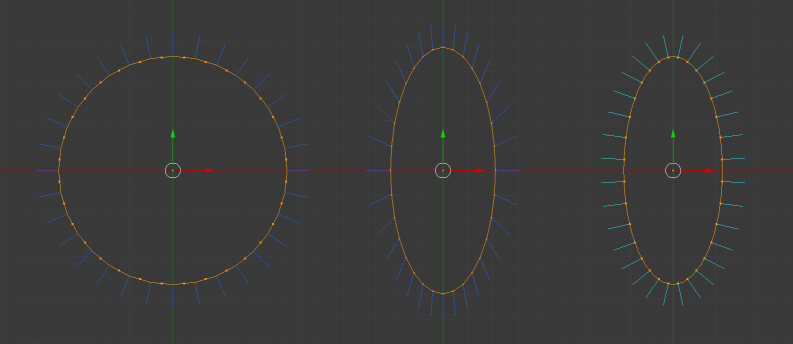# Baking Transformations

Usually, within Babylon.js, the translations, rotations and scaling of a mesh change its world matrix only and the vertex position data of a mesh is left unchanged. In certain situations you might be interested in applying a transform (position, rotation, scale) directly to the mesh vertices, instead of saving it as a the world matrix property of the mesh. This is called baking, and can be useful in the following situations:

• building a set of static geometry
• randomizing a series of mesh copies
• mirroring a mesh along an axis
• etc.

Any matrix can be used for this process including the current world matrix.

## Any Matrix

Using bakeTransformIntoVertices will bake the provided matrix directly into the mesh vertices changing their values but leaving the world matrix unchanged.

A box is created, rotated and positioned giving the following world matrix and vertex data.

``````World Matrix
-0.01    -0.01    1.00    0.00
-0.01     1.00    0.00    0.00
-1.00    -0.01  -0.01    0.00
1.00     2.00    3.00    1.00

Vertex Positions
1.00    -1.00     1.00
-1.00    -1.00     1.00
-1.00     1.00     1.00
1.00     1.00     1.00
1.00     1.00    -1.00
-1.00     1.00    -1.00
-1.00    -1.00    -1.00
1.00    -1.00    -1.00
``````

then bakeTransformIntoVertices is used with the world matrix

``````box.bakeTransformIntoVertices(box.getWorldMatrix());
``````

resulting in this world matrix and vertex data.

``````World Matrix
-0.01    -0.01    1.00    0.00
-0.01     1.00    0.00    0.00
-1.00    -0.01  -0.01    0.00
1.00     2.00    3.00    1.00

Vertex Positions
0.00    0.99    3.99
0.00    1.00    1.99
0.00    3.00    2.00
0.01    3.00    4.00
1.99    3.00    4.00
2.00    3.00    2.00
2.00    1.00    2.00
2.00    1.00    4.00
``````

Of course you do not have to use the world matrix, this code.

``````var matrix = BABYLON.Matrix.Scaling(1, -1, 1);
mesh.bakeTransformIntoVertices(matrix);
``````

will permanently mirror the mesh along the Y axis, while leaving the world matrix untouched.

## Current World Matrix

You can set the vertex positions based on any transformations that have been applied to a mesh and reset the world matrix to the identity matrix with bakeCurrentTransformIntoVertices.

A box is created, rotated and positioned giving the following world matrix and vertex data.

``````World Matrix
-0.01    -0.01    1.00      0.00
-0.01     1.00    0.00      0.00
-1.00    -0.01  -0.01      0.00
1.00     2.00    3.00      1.00

Vertex Positions
1.00    -1.00     1.00
-1.00    -1.00     1.00
-1.00     1.00     1.00
1.00     1.00     1.00
1.00     1.00    -1.00
-1.00     1.00    -1.00
-1.00    -1.00    -1.00
1.00    -1.00    -1.00
``````

then bakeCurrentTransformIntoVertices is applied to the box

``````box.bakeCurrentTransformIntoVertices();
``````

resulting in this world matrix and vertex data.

``````World Matrix
1.00    0.00    0.00    0.00
0.00    1.00    0.00    0.00
0.00    0.00    1.00    0.00
0.00    0.00    0.00    1.00

Vertex Positions
0.00    0.99    3.99
0.00    1.00    1.99
0.00    3.00    2.00
0.01    3.00    4.00
1.99    3.00    4.00
2.00    3.00    2.00
2.00    1.00    2.00
2.00    1.00    4.00
``````

## Use With Scaling

When baking scaling the normals are simply scaled in their current direction and so baking a scale can often give unrealistic results for lighting. To correct this normals need to be recomputed. This is illustrated in the following picture:In the above picture, you can see an untransformed mesh on the left, the same mesh with a baked scaling along the X axis in the middle and on the right, the mesh with its normals correctly recomputed.

You can do a recomputation of your normals like so:

``````var indices = mesh.getIndices();
var normals = mesh.getVerticesData(VertexBuffer.NormalKind);
BABYLON.VertexData.ComputeNormals(positions, indices, normals);
mesh.updateVerticesData(VertexBuffer.NormalKind, normals, false, false);
``````

Note: recomputing the normals of your mesh may not be an ideal solution, as the results may be wrong in some parts of the mesh (e.g. seams on a sphere).